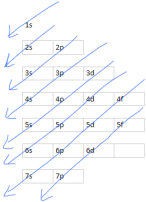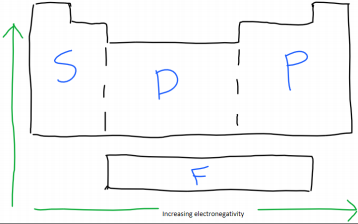×
Get Full Access to U of L - CHEM 101 - Study Guide - Midterm
Get Full Access to U of L - CHEM 101 - Study Guide - Midterm

×

U OF L / Chemistry / CHEM 101 / What is the configuration of an electron?

# What is the configuration of an electron? Description

##### Description: Sorry for the very late post, but here is what you should study for the upcoming exam. It accumulative so anything before this counts as well.
5 Pages 144 Views 1 Unlocks
Reviews

Chemistry study guide (exam 2)

## What is the configuration of an electron?∙ Electron configuration:

o Levels: numbers

o Sublevels: letters

o Orbital: A 3-d region where electrons can be found.

o Elements in the same group have the same number of valence electrons. o Valence electrons: Electrons on the outermost of the atom (valence shell),  that are eligible for bonding.

o The number of groups in a block determine how many max electrons the  level can have.

Sublevel

Max number of electrons

S

2

P

6

D

10

F

14

## What is the shorthand for electron configuration?Electron configuration chart

Example: Na has 11 electrons. What is the full electron configuration? Don't forget about the age old question of What is the meaning of conducting zone in the respiratory system?

∙ Following the electron configuration chart:

o 1s2 2s22p63s1

o You should always fill the level completely before moving on the next. If a  level is not completely filled at the end, that is ok.

## What is the electron configuration for ions?∙ Shorthand for electron configuration:

∙ You need to find the nearest noble gas before the element you're solving for. You  would not use Kr for Se because it comes after Se on the periodic table. So, the  noble gas must contain less electrons that the element you're solving for. ∙ Example:

o Br: [Ar] 4s2 3d10 4p6

o The [Ar] represents 1s2 2s2 2p63s23p6

o This is because Ar has 18 electrons and is able to represent the first 18 in Br.

∙ Electron configuration for ions:

∙ For positive ions, you will remove electrons.

∙ For negative ions, you will add electrons. Don't forget about the age old question of What does null mean in a hypothesis test?

∙ Ions the have the same number of electrons as another element, means that the two  are isoelectronic.

∙ Example:

o Na+

o The + represents the charge is now +1, so you will have to remove electrons. o Na = 11 electrons 11-1=10, so Na+has 10 electrons. If you want to learn more check out Where are alpha motor neurons located?

o 1s2 2s2 2p6

o Shorthand: [Ne]

o This means that Na+and Ne are isoelectronic. Don't forget about the age old question of What is it called when water vapor exit plant stomata?

∙ Covalent bonds:

∙ Nonpolar covalent: Bond between two non-metals that have the same  electronegativity and have equal sharing of electrons.

∙ Polar covalent: Between two non-metals with different electronegativity, which  causes the unequal sharing of electrons. The electrons will be displaced towards the  more electronegative atom. Don't forget about the age old question of What is breach of contract and what are the remedies for the breach of contract?

∙ Electronegativity: How willing an atom is to accept a pair of electrons.

∙ Lewis-dot structure:

∙ This is a representation of the bond between atoms/molecules.

2

∙ Octet rule: Valence shell can only contain 8 electrons and will maintain these 8 by  bonding with other atoms. When constructing a Lewis dot diagram, only 8  electrons are allowed to be represented per element.

∙ A single line represents 2 electrons ( - )

∙ A double line represents 4 electrons ( = )

∙ Molecular mass: Sum of all atomic mass' of each element in a covalently bonded  compound. We also discuss several other topics like What is the second stage of sleep?

∙ Formula mass: Sum of all atomic mass' of each element in an ionically bonded  compound.

∙ Finding molecular/formula mass:

∙ Example:

o H2O

▪ H- 2 x 1.008 = 2.02

▪ O- 1 x 16.00 = 16.00

▪ 2.02 + 16.00 = 18.02

o Because H has a subscript of 2, you multiply it's atomic mass by two.  (Because there are two atoms of this element) Because O has no subscript,  therefore only being one atom, it's atomic mass is multiplied by one.

∙ Percent composition:

∙ Percentage of mass in each element of a compound.

∙ You can simply change the amount in g to a percent by multiplying by 100. ∙ Example:

o 2.5g H and 7.5g C in a 10.0g sample.

▪ H- 2.5g H / 10.0g x 100 = 25%

▪ C- 7.5g C / 10.0g x 100 = 75%

∙ Percent composition with formula mass:

∙ Instead of dividing by the sample amount, you divide by the formula mass.

3

∙ Empirical formula:

∙ Lowest whole number ratio between elements in a compound.  o Simplified steps:

o Convert g to mols

o Divide all by lowest outcome

o Multiply if needed

∙ Example:

o 34.97g Fe / 55.85g Fe = .626 mol Fe

▪ Atomic mass

o 15.03g O / 16.00 g O = .939 mol O

o The smaller amount is .626, so all outcomes will be divided by that number. o .626 / .626 = 1

o .939 / .626 = 1.5

o We cannot use 1.5, so we must multiply to get a non-decimal number. o 1 x 2 = 2

o 1.5 x 2 = 3

o So the final answer will come out as Fe2O3

∙ Empirical formula from percent composition:

∙ Simplified steps:

o Convert % to grams

o Multiply if needed

∙ Molecular formula:

∙ Where empirical formula found the lowest whole number ratio, molecular formula  finds the actual whole number ratio.

o Molecular mass / Empirical formula mass = n

o N x Empirical formula mass = molecular formula

∙ Molarity:

∙ M = mol solute / L solution

∙ Example:

o 355mL contains .133 mols of sugar

4

o Convert mL to L (.335)

o .133 / .335 = .375M

∙ Solute: Smaller amount

∙ Solvent: Larger amount

∙ Solution: Solute & solvent

∙ Molarity = solution

∙ Dilution:

∙ M1 x V1 = M2 x V2

∙ Simplified steps:

o Identify what the question is asking for.

o Organize what is given.

o Plug and chug.

∙ The question will usually ask for one of the four elements listed in the first bullet.  The question should then give you the other three. After you have identified which  component you are looking for, arrange the equation to fit you need. For example,  if you need to find V1, arrange the equation to V1 = M2 x V2 / M1 . You put M1 on  the bottom because you are essentially dividing both sides of the equation by M1 to  get V1 by itself.This chart shows sublevel blocks and electronegativity on the periodic table.

5

Page ExpiredIt looks like your free minutes have expired! Lucky for you we have all the content you need, just sign up here